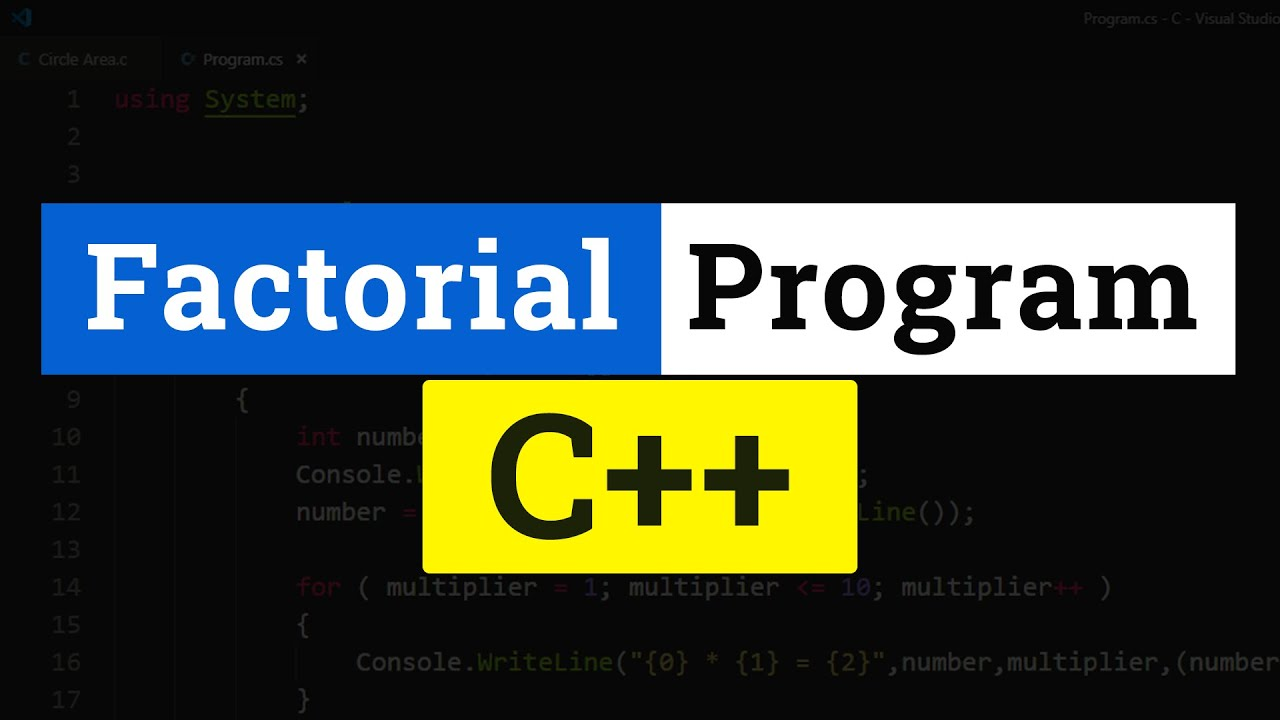# C++ Program to Find the Factorial of a Number TutorialIn this Tutorial you will learn to write a C++ Program to find the factorial of a number using Iterative Method ( for loop ).

In this Tutorial you will learn to write a C++ Program to find the factorial of a number using Iterative Method ( for loop ).

The factorial of a positive integer n, which is denoted as n!, is the product of all positive integers less than or equal to n.

That is n! = n * (n-1)(n-2)*....321

So 4! = 4 * 3 * 2 * 1 which is equal to 24.

Factorial for the numbers 0 and 1 is 1. That is 0! = 1 and 1! = 1. For negative numbers factorial value doesn't exists.

## Programming In Acceleration: Levelling Up Programming Skills

Some require and some are not. But acceleration programs might require you to build one. I’ll tell you how I made a computer program for the competition.

## Top 5 Programming Languages to Learn in 2021 | Top Programming Languages | Intellipaat

In this video, you will know the top 5 Programming languages to learn in 2021. It is always confusing for a beginner to choose a programming language from th...

## Coding 101: Programming Language Building Blocks

This article will introduce the concepts and topics common to all programming languages, that beginners and experts must know!

## C Programming for Beginners | C Programming Tutorial | Learn C Programming

C is an evergreen language and is widely used across different industries. This language is a must for students and working professionals to become good Software Engineer, especially when they are working in a Software Development Domain. Great Learning brings you this live session on ""C Programming for Beginners"". In this live session, we will be covering major concepts of C, starting with the syntax of the language. Then we will be covering topics such as comments, tokens, variables, etc. Then we will look at different data types and operators used for various mathematical operations, post which we will conclude our session with in-built libraries and conditional statements wherein we will talk about if, else if, nested if, and else block, and how multiple conditions can be replaced by a switch case. We will also understand the concepts of loops wherein we will talk about while loops, for loops and do-while loops including their similarities and differences.

## How to Create a Simple Calculator Program using C++ Programming Language ?

In this video tutorial you will learn How to create a simple Calculator Programming using C++ Programming Language.